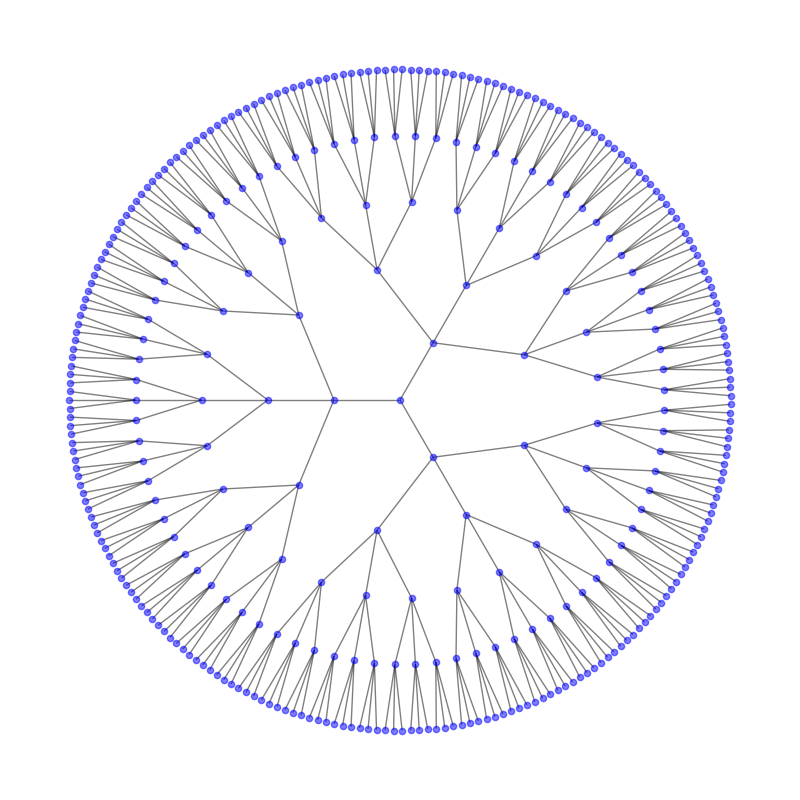# Circular Tree#

This example needs Graphviz and PyGraphviz.```import matplotlib.pyplot as plt
import networkx as nx

G = nx.balanced_tree(3, 5)
pos = nx.nx_agraph.graphviz_layout(G, prog="twopi", args="")
plt.figure(figsize=(8, 8))
nx.draw(G, pos, node_size=20, alpha=0.5, node_color="blue", with_labels=False)
plt.axis("equal")
plt.show()
```

Total running time of the script: ( 0 minutes 0.181 seconds)

Gallery generated by Sphinx-Gallery